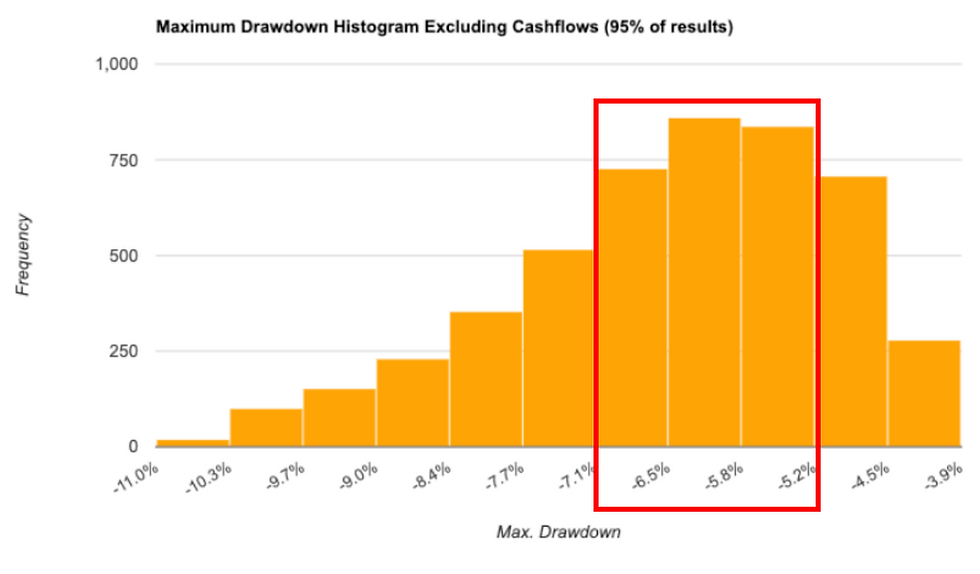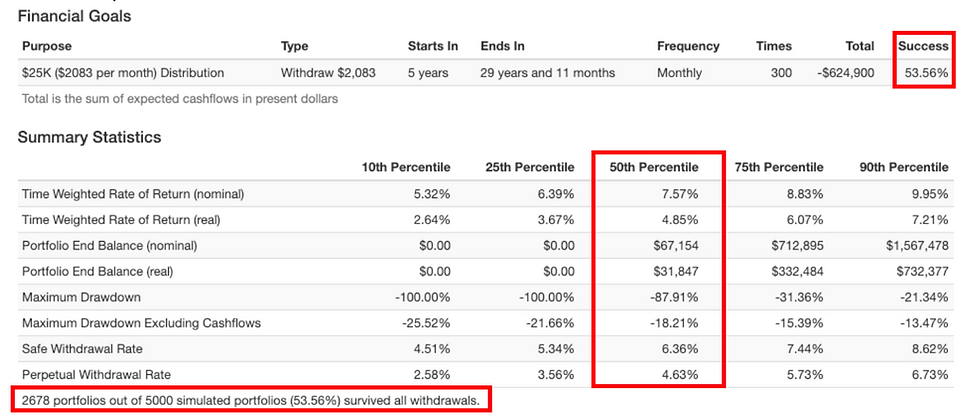top of page

# When will I be able to retire?Astoria has received a number of inquiries from financial advisors regarding the probability that their clients will be able to accomplish their retirement goals. As it is one of our firm’s unique skillsets, Astoria is able to leverage our quantitative capabilities, data, and technology to assess these probabilities. In this report, we utilize various Monte Carlo Simulations  to illustrate which scenario produces the highest probability of success for a hypothetical client’s retirement goals.

Inputs and Assumptions

• A client has \$300,000 in their retirement account and will invest in one of the following options for the next 5 years:

1. A portfolio of 20% stocks and 80% bonds

2. A portfolio of 40% stocks and 60% bonds

3. A portfolio of 60% stocks and 40% bonds

• Activate a distribution of \$25,000 (about \$2,083 per month) in 5 years

• Stay invested in each scenario for 30 years

Please note that past performance is not indicative of future results and results going forward will vary depending on returns of stocks and bonds going forward. For the purpose of these Monte Carlo Simulations, we are using the Statistical Returns Model  via our backtesting software, Portfolio Visualizer.

Investment Decision

Using the parameters highlighted above, the probability of success rises as we increase the percentage of stocks and decrease the percentage of bonds in the client’s portfolio. This is attributed to the greater returns that stocks have historically produced relative to bonds. Therefore, we would suggest the client invests his or her assets in a portfolio of 60% stocks and 40% bonds (Simulation C as seen below).

Alternatively, if the client’s tolerance for risk cannot be increased, we would suggest that the client withdraws less money per annum.

Simulation A – A portfolio of 20% stocks and 80% bonds 

• Simulation A initially invests the client’s assets in a portfolio of 10% US equities, 10% international equities, and 80% US bonds. After the distribution is initiated, we assume the client will thereafter remain in this portfolio.• Using the mean, volatility, and correlations of the selected asset classes, this simulation runs 5,000 portfolio scenarios in which 1,122 of those portfolios satisfy the client’s retirement goals. This represents a 22.44% probability of success. This is the lowest probability of success amongst the 3 simulations we performed.• The chart below displays inflation adjusted portfolio balances across the 10th, 25th, 50th, 75th, and 90th percentiles. The 90th percentile represents the highest extremes of portfolio balances while the 10th percentile represents the lowest extremes of portfolio balances over the 30-year investment period.• After year 17, the probability of success starts to decrease rapidly since the monthly withdrawal continues to reduce the value of the portfolio (see chart below).• At the end of year 5, the distribution activates and \$25,000 is withdrawn from the account each year until it is depleted entirely at the end of year 30. However, in year 26 the last withdrawal takes place and there is not enough money to continue the distribution in years 27-30 (see chart below).• As seen in the histogram below, the most frequently occurring end portfolio balance is \$0 (approximately 4,000 out of 5,000 occurrences). This intuitively makes sense since we are aiming to deplete the account.• As anticipated, the max portfolio drawdown including the cashflows resides between of 107% and 99% (approximately 3,900 out of 5,000 occurrences). This outcome is again attributed to the assumption that we are diminishing the account’s value at the end of the investment period (see chart below).• Conversely, the max portfolio drawdown excluding the cashflows resides between 5% and 7%. This is displayed in the histogram below.Simulation B – A portfolio of 40% stocks and 60% bonds 

• Simulation B initially invests the client’s assets in a portfolio of 20% US equities, 20% international equities, and 60% US bonds and reflects all the inputs and assumptions previously mentioned in Simulation A.

• This simulation produces a 42.46% probability of success (2,113 out of 5,000 portfolios), which is a greater than Simulation A. As mentioned previously, the more we increase the equity exposure, the higher the probability we can fulfill the client’s goal given stocks’ higher historical returns compared to bonds.• The probability of success starts to decrease after year 15. Note that the rate at which this probability decreases is less than that of Simulation A (see chart below).Simulation C – A portfolio of 60% stocks and 40% bonds 

• Simulation C initially invests the client’s assets in a portfolio of 30% US equities, 30% international equities, and 40% US bonds and reflects all the inputs and assumptions previously mentioned in Simulations A and B.

• This simulation produces a 53.56% probability of success (2,678 out of 5,000 portfolios) which is a greater than both Simulations A and B. As mentioned previously, the more we increase the equity exposure the higher the probability we can reach our goal given stocks’ higher historical return compared to bonds.• The probability of success starts to decrease after year 13. Note that the rate at which this probability decreases is meaningfully less than that of both Simulations A and B (see chart below).Conclusion

We are often asked by financial advisors, “What is the probability that my client will be able to retire?” As part of this process, we use a set of inputs from the advisor (current investment assets, withdrawal amount, time to retirement, current asset allocation mix, etc.) and use a quantitative statistical model (i.e. Monte Carlo Simulation), data, and technology to assign the probability that the end client’s goal can be accomplished.

In the example highlighted in this report, we recommend that the client increases their equity allocation to fulfill their retirement goals (Simulation C opposed to A & B). Otherwise, if the client’s tolerance for risk cannot be increased, we would suggest that the client withdraws less money per annum.

Best,

Nick Cerbone